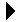Rational Number Package Body# Rational Number Package BodyRational Number Package Spec Download Rational Number Example Client```--
-- Body for rational number package.
--
with Gnat.Io; use Gnat.Io;
package body Rational_Number is
-- This is a private method to compute the GCD of two numbers using
-- Euclid's Algorithm.
function GCD(A, B: Integer) return Integer is
Ar: Integer := A;     -- These are the candidate values from the
Br: Integer := B;     --   algorithm.
ModVal: Integer;      -- Holds Ar mod Br.
begin
-- Algorithm repeats as long as you can create new Br values.  Stop
-- at zero when you cannot divide for next value.
while Br > 0 loop
-- Set for next iteration.
ModVal := Ar mod Br;
Ar := Br;
Br := ModVal;
end loop;

return Ar;
end GCD;

-- Use the GCD funtion to place two integers into lowest terms.
procedure Lowest(A, B: in out Unsigned) is
-- Remember the GCD.
The_GCD: Integer := GCD(A, B);
begin
A := A / The_GCD;
B := B / The_GCD;
end Lowest;

-- Return the rational in lowest terms.
function Lowest(R: Rational) return Rational is
-- Return value.
RetVal: Rational := R;
begin
-- Lower and send.
Lowest(RetVal.Num, RetVal.Denom);
return RetVal;
end Lowest;

-- Get there from an integer or pair of integers.
function To_Rational(Num: Integer; Den: Integer := 1) return Rational is
R: Rational;      -- Return value.
begin
-- Build and send.
R.Num := Num;
R.Denom := Den;
return R;
end To_Rational;

-- Output a rational.
procedure Put(R: Rational) is
-- Convert to lowest terms for printing.
RLow: Rational := Lowest(R);
begin
Put(RLow.Num);
if RLow.Denom /= 1 then
Put("/");
Put(RLow.Denom);
end if;
end Put;

-- This matches the denominators for addition and subtraction.  The two
-- functions are converted to have the same denominator.
procedure Common_Denom(A, B: in out Rational) is
-- The GCD of the denominators of A and B.

AMult: Integer;   -- Multiplier for A.
begin
-- Reduce the fractions and find the GCD..
A := Lowest(A);
B := Lowest(B);
GCD_Ad_Bd := GCD(A.Denom, B.Denom);

-- Re-write with a common denomiator.
AMult := B.Denom / GCD_Ad_Bd;             -- Multiplier for A.

A.Num := A.Num * AMult;                   -- Numerators.
B.Num := B.Num * (A.Denom / GCD_Ad_Bd);

A.Denom := A.Denom * AMult;               -- Denominators.
B.Denom := A.Denom;
end Common_Denom;

-- Four functions.
function "+" (A, B: Rational) return Rational is
-- Copies of the args so we can change them.
ACopy: Rational := A;
BCopy: Rational := B;
begin
Common_Denom(ACopy, BCopy);
end "+";

function "-" (A, B: Rational) return Rational is
-- Copies of the args so we can change them.
ACopy: Rational := A;
BCopy: Rational := B;
begin
Common_Denom(ACopy, BCopy);
end "-";

function "*" (A, B: Rational) return Rational is
-- Copies of the args so we can change them.
ACopy: Rational := Lowest(A);
BCopy: Rational := Lowest(B);
begin
-- These changes preserve the product and can prevent overflow under
-- some circumstances.
Lowest(ACopy.Num, BCopy.Denom);
Lowest(BCopy.Num, ACopy.Denom);

-- Make the answer.
return To_Rational(ACopy.Num * BCopy.Num, ACopy.Denom * BCopy.Denom);
end "*";

function "/" (A, B: Rational) return Rational is
begin
return A * To_Rational(B.Denom, B.Num);
end "/";

-- Equality test.  Sees if they are the same after reduction to
-- lowest terms.
function "=" (A, B: Rational) return Boolean is
ALow: Rational := Lowest(A);
BLow: Rational := Lowest(B);
begin
return ALow.Num = BLow.Num and ALow.Denom = BLow.Denom;
end "=";

end Rational_Number;
```Rational Number Package Spec Rational Number Example Client